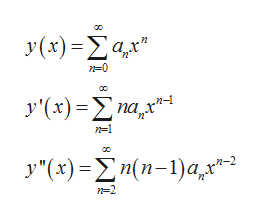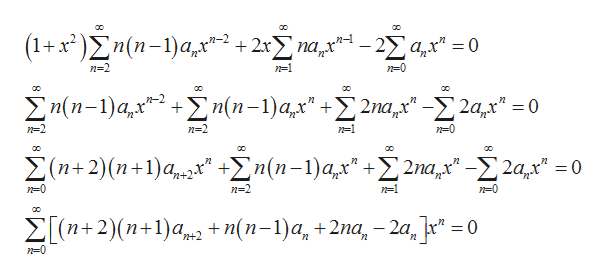2

Question

2

Step 1

From the given information it is needed to calculate the initial value problem using power series for the problem:

Step 2

The power series solution and its derivative to above initial value problem is:help_outlineImage Transcriptionclosey(1) -Σα ο y(x) Σa,r" y'(x) = Ση(n-1) a,r"1 -n-2 n-2 8 fullscreen
Step 3

Now, put these values into the given dif...help_outlineImage Transcriptioncloseσο 3α (1+x)Ση(n - 1) a,r" + 2xΣ mr-2Σ α" -0 n-2 n1 Σ/n-1)a, Zn(п-1)а,x + Σ/n-1) aχ" +Σ Σu" -Σ 2αμ. 2ах" 3D0 n=2 n-2 n1 n0 ο σ ο Σ (n+2) (n+ 1) a,,x" +Ση (n -1) a, ά σο 2па,х" 2а,х" %3D0 n-0 n-2 n-1 n-0 oο Σ(n+2)(n+ 1) α,, + n(n-1) α, + 2na,-2α,-0 n-0 fullscreen

Want to see the full answer?

See Solution

Want to see this answer and more?

Our solutions are written by experts, many with advanced degrees, and available 24/7

See Solution
Tagged in

Math